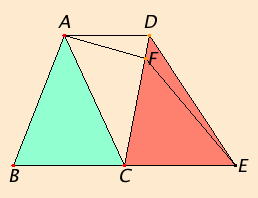# Proposition 40

Equal triangles which are on equal bases and on the same side are also in the same parallels.

Let ABC and CDE be equal triangles on equal bases BC and CE and on the same side.I say that they are also in the same parallels.

I.Post.1

Join AD. I say that AD is parallel to BE.

If not, draw AF through A parallel to BE, and join FE.

I.38

Therefore the triangle ABC equals the triangle FCE, for they are on equal bases BC and CE and in the same parallels BE and AF.

C.N.1

But the triangle ABC equals the triangle DCE, therefore the triangle DCE also equals the triangle FCE, the greater equals the less, which is impossible. Therefore AF is not parallel to BE.

Similarly we can prove that neither is any other straight line except AD, therefore AD is parallel to BE.

Therefore equal triangles which are on equal bases and on the same side are also in the same parallels.

Q.E.D.

## Guide

The statement and the setting out of this proposition are not up to Euclid’s standards. This is supposed to be a converse to I.38. The statement should state the the bases are along the same line, but it doesn’t. There is no justification for assuming that the point C is a common vertex of the two triangles. Fortunately, the proof works just as well if C is split into two points.

For some of the propositions and many of the lemmas and corollaries in the Elements, there is evidence that Euclid did not write them, but they were added later. The process of incorporating new material in textbooks was almost automatic when the books were copied by hand instead of printed. Scholars wrote comments (called “scholia”, singular “scholium”) in the margins of the texts, and copyists (some of whom were later scholars) would include those comments as part of the text in their new copies.

Heiberg could show by means of an early papyrus fragment that this proposition was an early interpolation. For others, such as I.37 there is no direct evidence, only a doubt that a mathematician of Euclid’s caliber would have included them.

Unlike the other propositions in Book I, this one is not used later in the Elements.